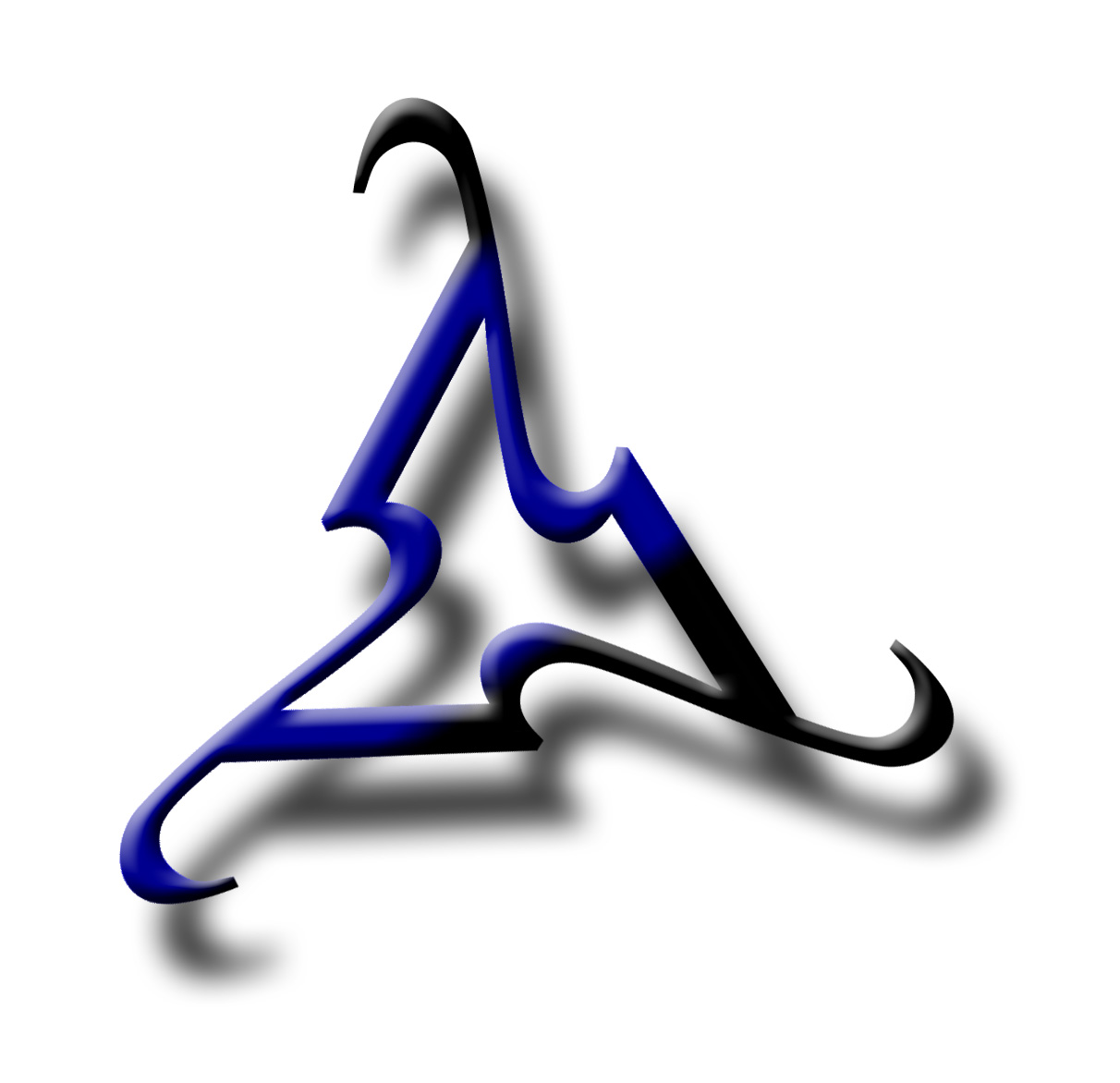Introduction to Logic ToolsforThought

 Rotors

A natural number n is a rotor if and only if the decimal representation of n+n is the same as a rotation of the decimal representation of n in which the last digit has been moved to the front. In other words, the decimal representation n1 n2 ... nk-1 nk satisfies the following sum.

 n1 n2 ... nk-1 nk + n1 n2 ... nk-1 nk nk n1 n2 ... nk-1

What is the smallest rotor? (Hint: It is a big number.)